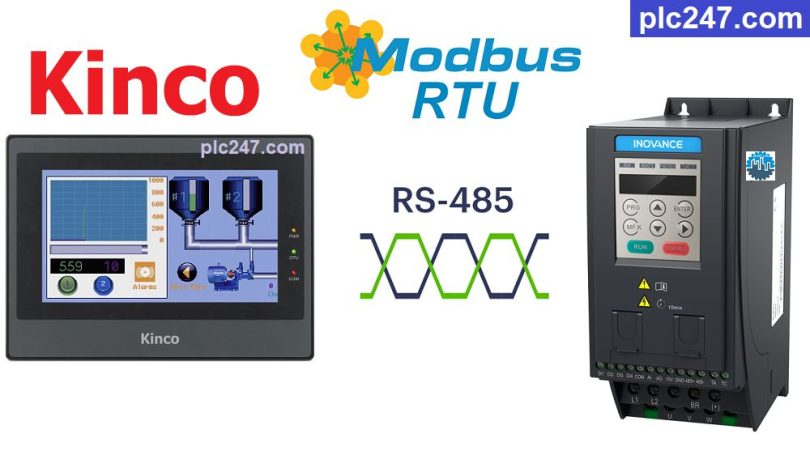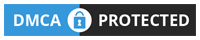# HMI Kinco “Modbus RTU” Inovance MD200 TutorialWritten by

Hello friends!
Kinco HMI is a low-cost but extremely high-quality HMI, it supports all the basic connection types that an HMI needs such as: RS232, RS422/485, Ethernet and including “Modbus RTU”

Today plc247.com will guide you to use the Kinco MT4414TE HMI to control the Inovance MD200 inverter through the Modbus RTU protocol.
Please note that the Kinco HMI and the Omron HMI (NB Series) have the same hardware and software, so with the Omron HMI we can do the same.

#### HMI Kinco & Inovance MD200 “Wiring Diagram”

+ Below is the RS485 communication wiring diagram between the Kinco HMI and the Inovance MD200

#### Inovance MD200 Modbus Parameters Setting

In addition to the basic parameters of the inverter installed according to the motor (frequency, current, voltage…) we need to install the following communication parameters to be able to control the Inovance MD200 with Modbus RTU.

##### Note: HMi Kinco Registers Address = “VFD Address (Dec) + 1“

>>> VFD Control Address = 2000 (hex) = 8192 (dec) + 1 = 8193 (dec)

• Set Word 8193 = 1 >>> Forward Run
• Set Word 8193 = 2 >>> Reverse Run
• Set Word 8193 = 6 >>> Stop

>>> Set Frequency Address = 1000 (hex) = 4096 (dec) + 1 = 4097 (dec)
>>> Frequency Reference = (0 to 10000 dec) ~ (0 to VFD Max Frequency)

+ Output Frequency Address = 1001 (hex) = 4097 (dec) + 1 = 4098 (dec)
Output Voltage Address = 1003 (hex) = 4099 (dec) + 1 = 4100 (dec)
Output Current Address = 1004 (hex) = 4100 (dec) + 1 = 4101 (dec)

#### HMI Kinco “Modbus RTU” Configuration

To be able to control the Inovance MD200 using Modbus protocol, we need to set the communication parameters between the two devices to be the same:

### HMI Kinco Modbus Example

+ Control Panel Design

+ Frequency Setting

+ Forward Run

+ Reverse Run

+ Stop Command

### Project Video Tutorial

======

##### References